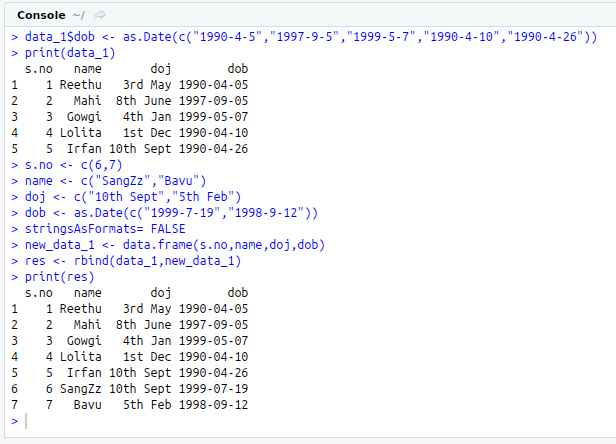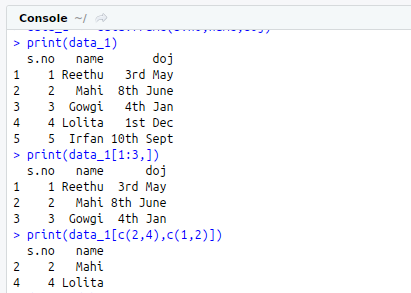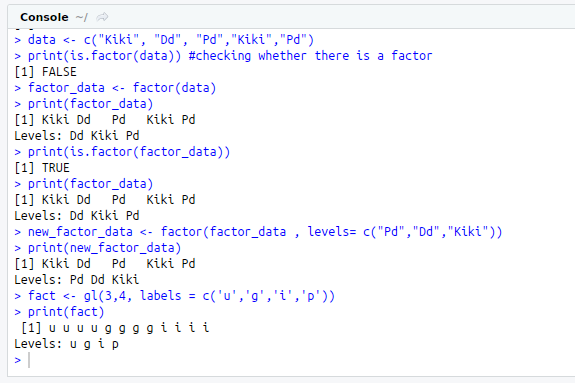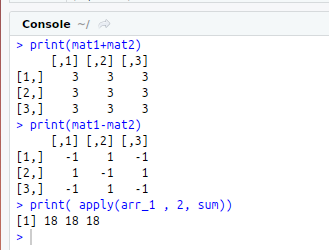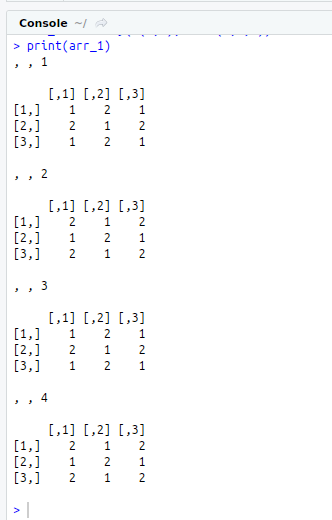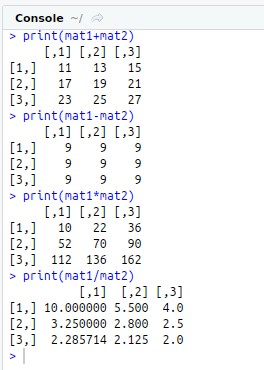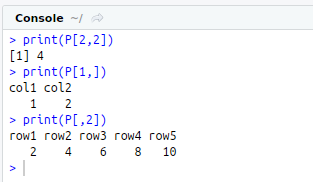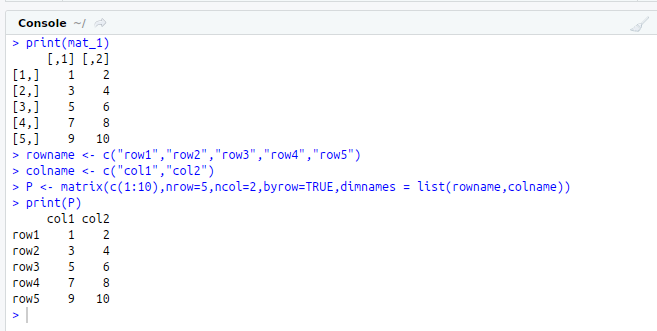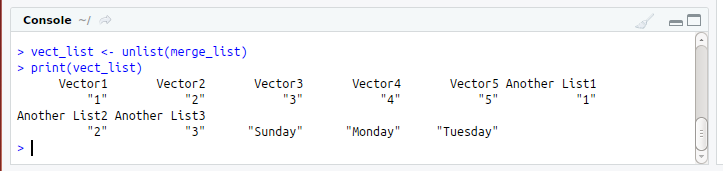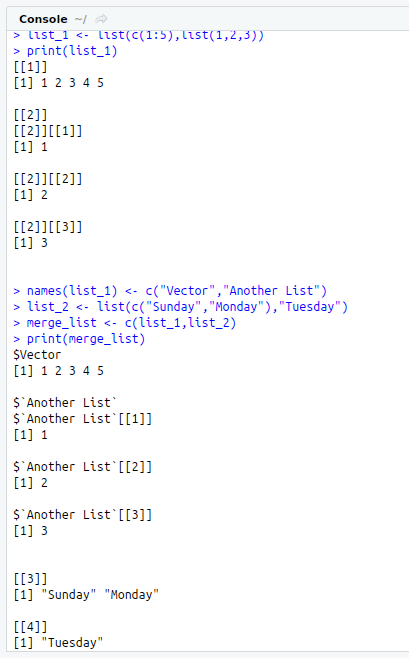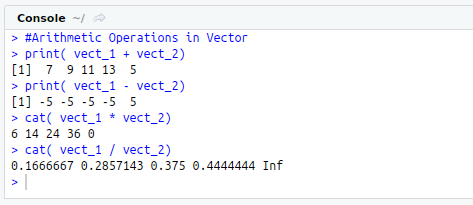• #5, First Floor, 4th Street Dr. Subbarayan Nagar Kodambakkam, Chennai-600 024 Landmark : Samiyar Madam
• pro@slogix.in
• +91- 81240 01111

What are the Data types in R?

Description

To know about the data types in R programming.

Process
• In computing, data is information that has been translated into a form that is efficient for movement or processing.
• Data is available as a raw value.

• Vectors
• Lists
• Matrices
• Arrays
• Factors
• Data Frames

1) Vector :

• In R programming, the very basic data types are the R-objects called vectors which hold elements of different classes.
• Types–integer, numeric, logical, character, complex
• R Function : c()

2) List:

• A List can contain vector, function and even another list inside it.
• R Function : list()

3) Matrices:

• Matrix is a two-dimensional rectangular data set.
• R Function : matrix()

4) Array:

• While matrices are confined to two dimensions, arrays can be of any number of dimensions.
• R Function : array()

5) Factors:

• Factors are created using vectors.
• It stores the vector along with the distinct values of the elements in the vector as labels.
• R Function : factor()

6) Data Frames:

• Data frames are tabular data objects.
• It is a list of vectors of equal length.
• R Function : data.frame()
Sapmle Code

#VECTOR
#Create two vector
vect_1<-c(1,2,3,4,5)
vect_2<-c(6,7,8,9,0)

#Arithmetic Operations in Vector
print( vect_1 + vect_2)
print( vect_1 – vect_2)
cat( vect_1 * vect_2)
cat( vect_1 / vect_2)

#LIST
#Create a list
list_1<-list(c(1:5),list(1,2,3))
print(list_1)
#Give names to the elements in the list
names(list_1)<-c(“Vector”,”Another List”)
#Creating another list
list_2<-list(c(“Sunday”,”Monday”),”Tuesday”)
#Merging two list
merge_list<-c(list_1,list_2)
print(merge_list)
#Converting list into vector
vect_list<-unlist(merge_list)
print(vect_list)

#MATRIX
#Create a matrix
mat_1<-matrix(c(1:10),nrow=5,ncol=2,byrow=TRUE)
print(mat_1)
#GIve names to the matrix rows and columns
rowname<-c(“row1″,”row2″,”row3″,”row4″,”row5”)
colname<-c(“col1″,”col2”)
P<-matrix(c(1:10),nrow=5,ncol=2,byrow=TRUE,dimnames = list(rowname,colname))
print(P)
#Printing the element in 2nd row and 2nd column
print(P[2,2])
#Printing the first row
print(P[1,])
#Printing the second column
print(P[,2])

#Arithmetic Operations in matrix
mat1<-matrix(c(10:18),nrow=3,byrow=TRUE)
mat2<-matrix(c(1:9),nrow=3,byrow=TRUE)
print(mat1+mat2)
print(mat1-mat2)
print(mat1*mat2)
print(mat1/mat2)

#ARRAY
#Create an array
arr_1<-array(c(1,2),dim=c(3,3,4))
print(arr_1)

#Arithmetic Operation in array
mat1<-arr_1[,,1]
mat2<-arr_1[,,2]
print(mat1+mat2)
print(mat1-mat2)
#apply function
print( apply(arr_1 , 2, sum))

#FACTORS
#Create a factor
data<-c(“Kiki”, “Dd”, “Pd”,”Kiki”,”Pd”)
print(is.factor(data)) #checking whether there is a factor
factor_data<-factor(data)
print(factor_data)
print(is.factor(factor_data))
#Changing the Order of the Levels
print(factor_data)
new_factor_data<-factor(factor_data , levels= c(“Pd”,”Dd”,”Kiki”))
print(new_factor_data)
#Generating Factor Level
fact<-gl(3,4, labels = c(‘u’,’g’,’i’,’p’))
print(fact)

#DATA FRAME
#Create a data frame
s.no<-c(1:5)
name<-c(“Reethu”,”Mahi”,”Gowgi”,”Lolita”,”Irfan”)
doj<-c(“3rd May”,”8th June”,”4th Jan”,”1st Dec”,”10th Sept”)
stringsAsFactors=FALSE
data_1<-data.frame(s.no,name,doj)
print(data_1)
#Printing first to third row
print(data_1[1:3,])
#Printing 2nd,4th row and 1st,2nd column
print(data_1[c(2,4),c(1,2)])
#Adding Columns to the existing data frame
data_1\$dob<-as.Date(c(“1990-4-5″,”1997-9-5″,”1999-5-7″,”1990-4-10″,”1990-4-26”))
print(data_1)
#Adding Rows to the Existing data frame
s.no<-c(6,7)
name<-c(“SangZz”,”Bavu”)
doj<-c(“10th Sept”,”5th Feb”)
dob<-as.Date(c(“1999-7-19″,”1998-9-12”))
stringsAsFormats= FALSE
new_data_1<-data.frame(s.no,name,doj,dob)
res<-rbind(data_1,new_data_1)
print(res)

Screenshots